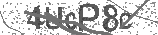﻿ The Adding by 1 Song (Math Facts) - Addition Song for Kids | Silly School Songs | Safe Videos for Kids
Welcome

# The Adding by 1 Song (Math Facts) - Addition Song for Kids | Silly School Songs

Thanks! Share it with your friends!

URL

You disliked this video. Thanks for the feedback!

Sorry, only registred users can create playlists.URLFind Related Videos  added
58 Views## Description

This fun addition song for kids teaches how to add by 1 up to 10, both forwards and backwards. The rhythm supports long-term memory development of the math facts. Enjoy!

For more music and information, please visit http://sillyschoolsongs.com.

Lyrics:

It's time to learn our math facts!

1 + 0 = 1
1 + 1 = 2
1 + 2 = 3
1 + 3 = 4
1 + 4 = 5
1 + 5 = 6
1 + 6 = 7
1 + 7 = 8
1 + 8 = 9
1 + 9 = 10

Alright, great job!

9 + 1 = 10
8 + 1 = 9
7 + 1 = 8
6 + 1 = 7
5 + 1 = 6
4 + 1 = 5
3 + 1 = 4
2 + 1 = 3
1 + 1 = 2
0 + 1 = 1

Alright, let's do it again
And go all the way up,
Then all the way back down.

1 + 0 = 1
1 + 1 = 2
1 + 2 = 3
1 + 3 = 4
1 + 4 = 5
1 + 5 = 6
1 + 6 = 7
1 + 7 = 8
1 + 8 = 9
1 + 9 = 10
9 + 1 = 10
8 + 1 = 9
7 + 1 = 8
6 + 1 = 7
5 + 1 = 6
4 + 1 = 5
3 + 1 = 4
2 + 1 = 3
1 + 1 = 2
0 + 1 = 1

Well, that's a wrap, kids!
You did a fantastic job.
We'll see you next time!Be the first to comment